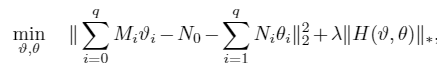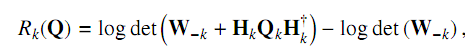# Unable to minimize sum of two convex functions: Unsolved

The objective function I aim to minimize isIn the above equation M_i and N_i are constant matrices. H is a matrix which is affine in v and \theta. The expression is convex. When I try to solve using CVX, I get the error message: "Too many output arguments"

However, when I solve either
\begin{equation}
\underset{v,\theta}{\text{ min } } ||\sum\limits_{i=0}^{q} M_{i}v_{i} - N_{0} - \sum\limits_{i=1}^{q} N_{i}\theta_{i}||_{2}^{2}
\end{equation}

Or, if I solve
\begin{equation}
\underset{v,\theta}{\text{ min } } \lambda ||H(v,\theta)||_{*} \text{ : the nuclear norm}
\end{equation}
separately I am able to get feasible solutions.

What am I doing wrong when I combine the two functions together? I want to also mention that this optimization problem aims at giving me \theta , v for an over-determined set of equation actually.
Solver used : SDPT3 (because that is the solver used in the literature that I refer)

Thank you, I hope I could get some support as I do not understand the error message. I would like to get it clear if the error arises because of the formulation of the problem or is it something with CVX actually.

I know for sure the objective is Convex and in the literature I refer they have also used CVX to solve this optimization problem.

Meeting the same problem with you recently！What I try to optimize is the subtraction of two concave functions.then the MATLAB give the result as following:
Error using cvx/plus (line 83)
Disciplined convex programming error:
Illegal operation: {concave} - {concave}

Error in cvx/minus (line 21)
z = plus( x, y, true, cheat );

Error in antenna_cvx_tool (line 41)
B(iUser)=log_det(Wk+w_all(:,:,iUser))-log_det(Wk);

I just don’t find out where the problem is!Have you solved yet?